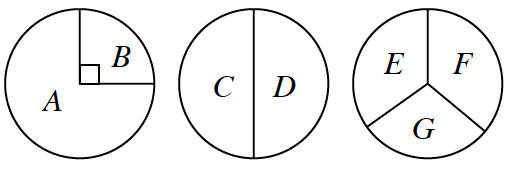### Home > CCG > Chapter Ch8 > Lesson 8.3.3 > Problem8-127

8-127.

Three spinners are shown at right. If each spinner is randomly spun and if spinners #2 and #3 are each equally divided, find the following probabilities.

1. $P(\text{spinning } A, C, \text{ and } E)$

2. $P(\text{spinning at least one vowel})$

Use a tree diagram to illustrate all possible combinations that can occur if each spinner is spun once.
Refer to the Math Notes box in Lesson 4.2.3 for information on tree diagrams.